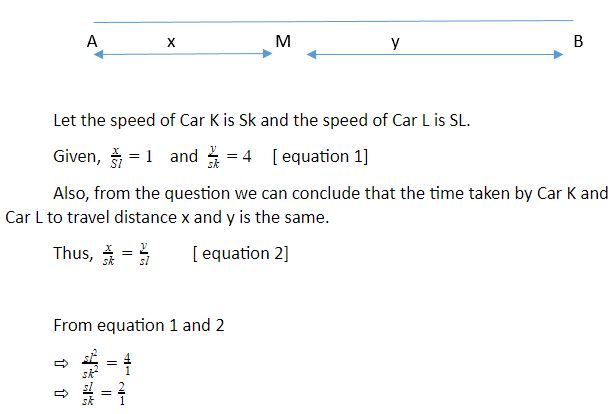# Explanation

Answer    Let the distance from A to M is x and the distance from B to M is y.From above we can conclude that the speed of Car L is twice the speed of Car k. Thus for the same distance travelled, the time taken by Car L is half the time taken by Car K.

Total Time taken by Car L = ½ (time taken by Car K to travel y distance) + Time taken by Car K to travel x distance.

• Total time by Car L = 2 + 1 = 3 hours.